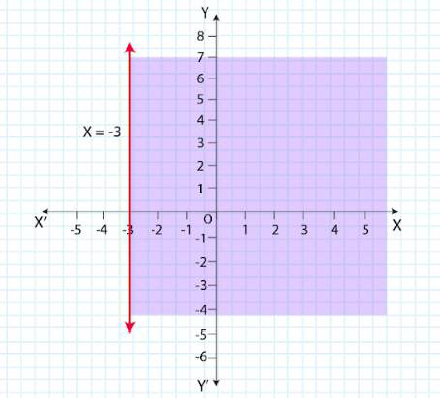# NCERT Solutions For Class 11 Math Chapter – 6 Exercise – 6.2

NCERT Solutions For Class 11 Math Chapter – 6 Exercise – 6.2

Solve the following inequalities graphically in two-dimensional plane:

1. x + y < 5

Solution:

Given x + y < 5

Consider

 X 0 5 y 5 0

Now draw a dotted line x + y = 5 in the graph (∵ x + y = 5 is excluded in the given question)

Now Consider x + y < 5

Select a point (0, 0)

⇒ 0 + 0 < 5

⇒ 0 < 5 (this is true)

∴ Solution region of the given inequality is below the line x + y = 5. (That is origin is included in the region)

The graph is as follows: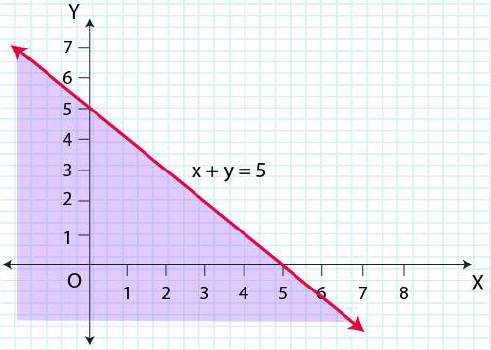2. 2x + y ≥ 6

Solution:

Given 2x + y ≥ 6

Now draw a solid line 2x + y = 6 in the graph (∵2x + y = 6 is included in the given question)

Now Consider 2x + y ≥6

Select a point (0, 0)

⇒ 2 × (0) + 0 ≥ 6

⇒ 0 ≥ 6 (this is false)

∴ Solution region of the given inequality is above the line 2x + y = 6. (Away from the origin)

The graph is as follows: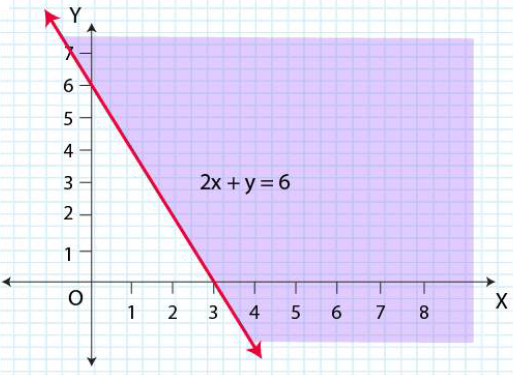3. 3x + 4y ≤ 12

Solution:

Given 3x + 4y ≤ 12

Now draw a solid line 3x + 4y = 12 in the graph (∵3x + 4y = 12 is included in the given question)

Now Consider 3x + 4y ≤ 12

Select a point (0, 0)

⇒ 3 × (0) + 4 × (0) ≤ 12

⇒ 0 ≤ 12 (this is true)

∴ Solution region of the given inequality is below the line 3x + 4y = 12. (That is origin is included in the region)

The graph is as follows: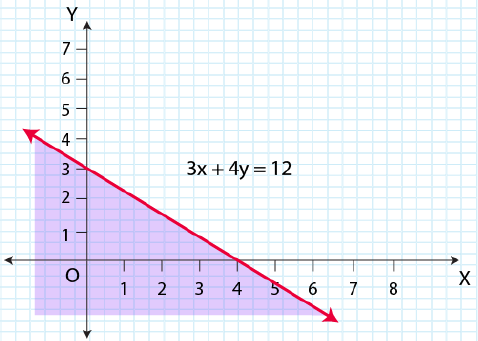4. y + 8 ≥ 2x

Solution:

Given y + 8 ≥ 2x

Now draw a solid line y + 8 = 2x in the graph (∵y + 8 = 2x is included in the given question)

Now Consider y + 8 ≥ 2x

Select a point (0, 0)

⇒ (0) + 8 ≥ 2 × (0)

⇒ 0≤ 8 (this is true)

∴ Solution region of the given inequality is above the line y + 8 = 2x. (That is origin is included in the region)

The graph is as follows: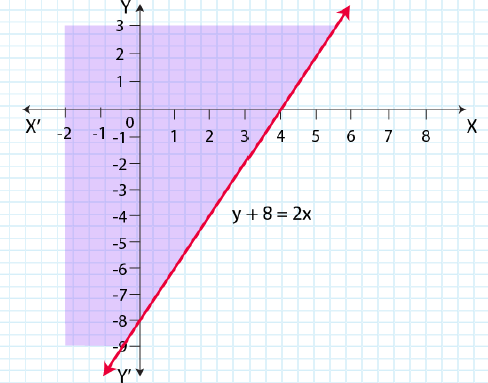5. x – y ≤ 2

Solution:

Given x – y ≤ 2

Now draw a solid line x – y = 2 in the graph (∵ x – y = 2 is included in the given question)

Now Consider x – y ≤ 2

Select a point (0, 0)

⇒ (0) – (0) ≤ 2

⇒ 0 ≤ 2 (this is true)

∴ Solution region of the given inequality is above the line x – y = 2. (That is origin is included in the region)

The graph is as follows: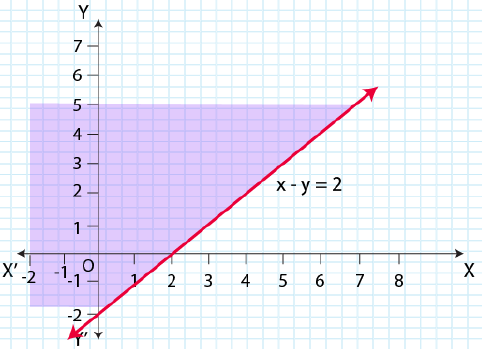6. 2x – 3y > 6

Solution:

Given 2x – 3y > 6

Now draw a dotted line 2x – 3y = 6 in the graph (∵2x – 3y = 6 is excluded in the given question)

Now Consider 2x – 3y > 6

Select a point (0, 0)

⇒ 2 × (0) – 3 × (0) > 6

⇒ 0 > 6 (this is false)

∴ Solution region of the given inequality is below the line 2x – 3y > 6. (Away from the origin)

The graph is as follows: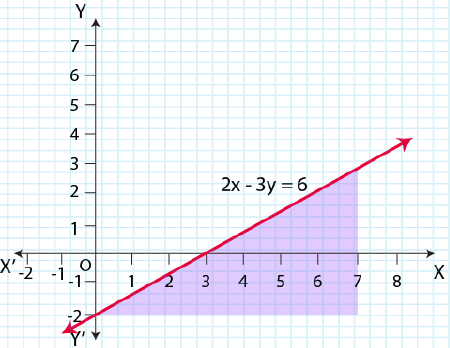7. – 3x + 2y ≥ – 6

Solution:

Given – 3x + 2y ≥ – 6

Now draw a solid line – 3x + 2y = – 6 in the graph (∵– 3x + 2y = – 6 is included in the given question)

Now Consider – 3x + 2y ≥ – 6

Select a point (0, 0)

⇒ – 3 × (0) + 2 × (0) ≥ – 6

⇒ 0 ≥ – 6 (this is true)

∴ Solution region of the given inequality is above the line – 3x + 2y ≥ – 6. (That is origin

is included in the region)

The graph is as follows: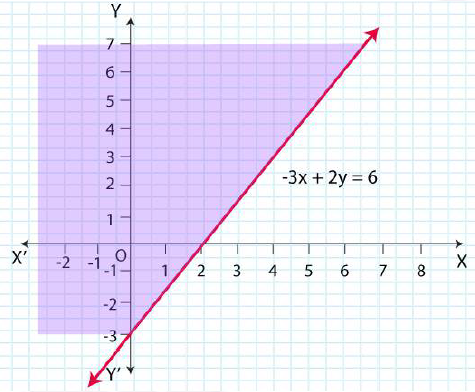8. y – 5x < 30

Solution:

Given y – 5x < 30

Now draw a dotted line 3y – 5x = 30 in the graph (∵3y – 5x = 30 is excluded in the given question)

Now Consider 3y – 5x < 30

Select a point (0, 0)

⇒ 3 × (0) – 5 × (0) < 30

⇒ 0 < 30 (this is true)

∴ Solution region of the given inequality is below the line 3y – 5x < 30. (That is origin is included in the region)

The graph is as follows: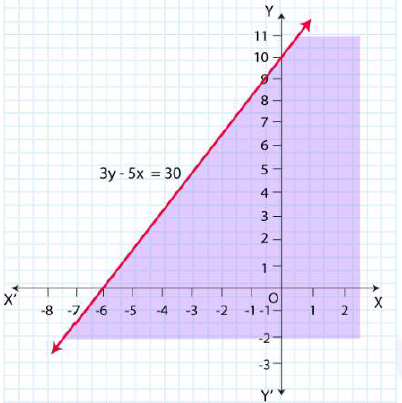9. y < – 2

Solution:

Given y < – 2

Now draw a dotted line y = – 2 in the graph (∵ y = – 2 is excluded in the given question)

Now Consider y < – 2

Select a point (0, 0)

⇒ 0 < – 2 (this is false)

∴ Solution region of the given inequality is below the line y < – 2. (That is Away from the origin)

The graph is as follows: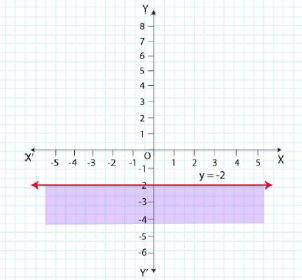10. x > – 3

Solution:

Given x > – 3

Now draw a dotted line x = – 3 in the graph (∵x = – 3 is excluded in the given question)

Now Consider x > – 3

Select a point (0, 0)

⇒ 0 > – 3

⇒ 0 > – 3 (this is true)

∴ Solution region of the given inequality is right to the line x > – 3. (That is origin is included in the region)

The graph is as follows: